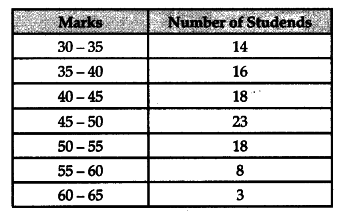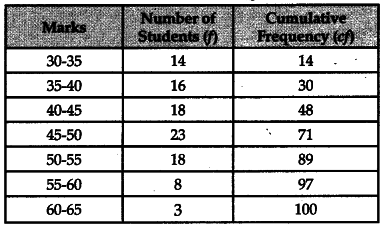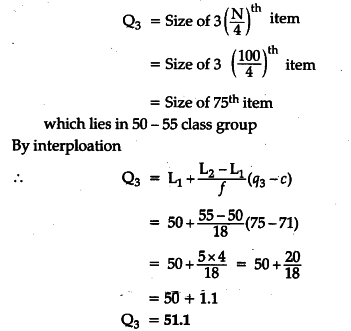# Calculate the value of median

Calculate the value of median, first quartile (Q1), and third quartile (Q3)for the following data :Calculation of First and Third Quantile and Median: